Materials Square
MatSQ Docs - Data (en)
The Data page lists all calculations and provides the original data files of the calculations.Successfully finished (id : jobID)

A direct connection to the 'Data' page is enabled from the status bar of the 'Simulation' module. The numbers shown in the parentheses is the job id, which is the unique ID of a job, and you can click on a job id to see its raw data of the calculation.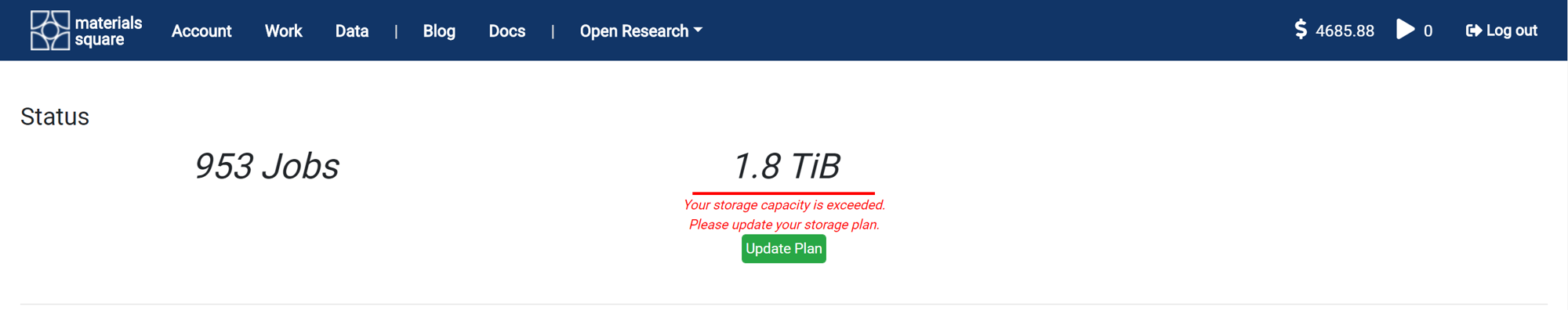It shows the total number of jobs performed so far and the occupied storage capacity. The default storage capacity is 100 GB. If you need it, you can store more data in the cloud by purchasing a storage plan.

The output of a simulated computation is in the text form by default. If the module does not load the desired data, you can locate the desired data by reading the original data.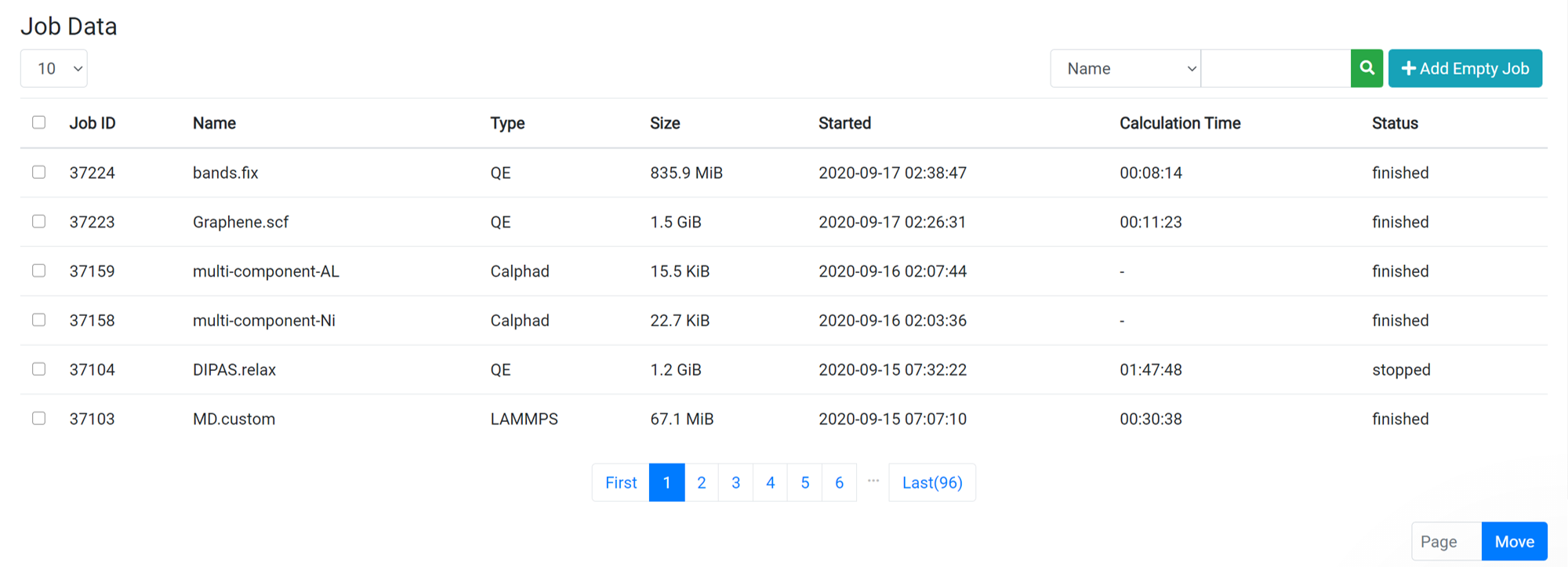When you click on one of the jobs from the job list on the Data page, the raw data file list is displayed at the same time the Parent Work information is displayed. For information about the files that appear, see the following File Types and Description: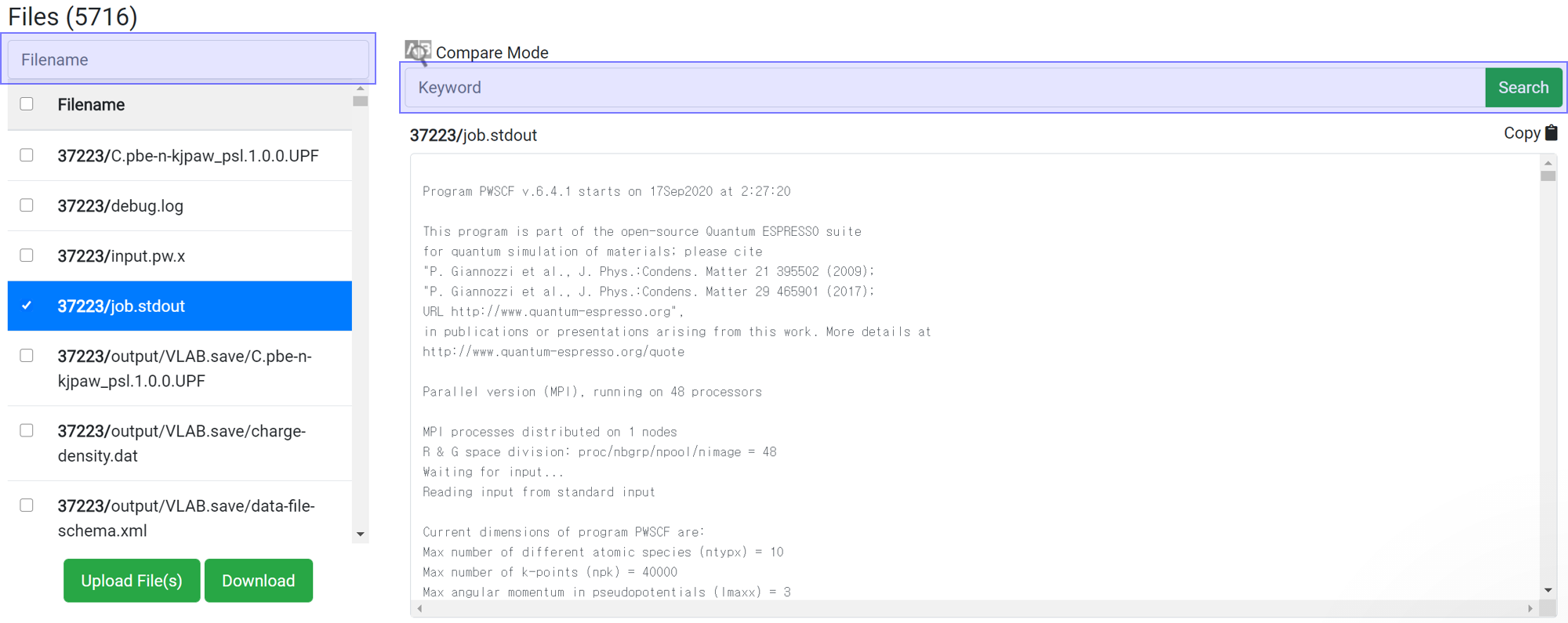Search enables to show only the desired files or information. This search box does the same function as the 'grep' command in Linux. This search box is case sensitive.

File Location File Name Description
input.pw.x The input script of a PWscf (pw.x) calculation.
job.stdout The output file of a PWscf (pw.x) calculation containing all data related to the basic calculation.
job.stdout.pp.x The output file of a Charge Density (pp.x) calculation.
job.stdout.projwfc.x The output file of a DOS (projwfc.x) calculation.
job.stdout.bands.x The output file of a Band Structure (bands.x) output calculation.
*.UPF The pseudopotential file used in calculations.
output/ VLAB.xml The file containing the same data as job.stdout.
output/ VLAB.wfc* The files contain the wavefunction data. It is a large file and is in an unreadable binary format.
output/VLAB.save/ charge-density.dat The file containing the charge density.
output/VLAB.save/ data-file-schema.xml The file containing the same data as job.stdout.
output/VLAB.save/ paw.txt The file created for PAW pseudopotential.
output/VLAB.save/ wfc*.dat The files contain the wavefunction data. It is a large file and is in an unreadable binary format.
vlab/trajectory/ *.dat When you perform calculations with multiple scf steps or molecular dynamics calculations, this file saves the movement of the each atom for the snap shots in the trajectory.
vlab/trajectory/ msd The file containing the msd data.
pp.x/ input.pp.x The input script file of charge density (pp.x) calculations.
pp.x/ ppoutput.* The output script file of charge density (pp.x) calculations.
pp.x/ *.cube The file containing the results corresponding to the plot number selected in charge density (pp.x) calculations.
pp.x/ ACF.dat It contains atom order number, the coordinates of each atom, the charge associated with it according to Bader partitioning, percentage of the whole according to Bader partitioning and the minimum distance to the surface.
pp.x/ AVF.dat The number of each volume that has been assigned to each atom.
pp.x/ BCF.dat It contains the coordinates of each Bader maxima, the charge within that volume, the nearest atom and the distance to that atom.
pp.x/ avg.dat The output file of the Macroscopic average. The 1, 2, 3 are the results that correspond to the x, y, z-axis.
pp.x/ input.pp.x.avg.in The input file for the Macroscopic average calcluation.
pp.x/ ppoutput.bader.* The output file of Bader charge calculation using the charge density.
bands.x/ input.bands.x The input script file of band structure (bands.x) calculations.
bands.x/ bandsx The file containing information on each band.
bands.x/ bandsx.gnu A gnu file for drawing a band structure graph.

The most important file to check is job.stdout. Most of the output information calculated by Quantum Espresso is written to job.stdout. Therefore, checking this file can yield a lot of data, such as checking energy in the middle of a calculation, verifying convergence, estimating the estimated calculation time, and more.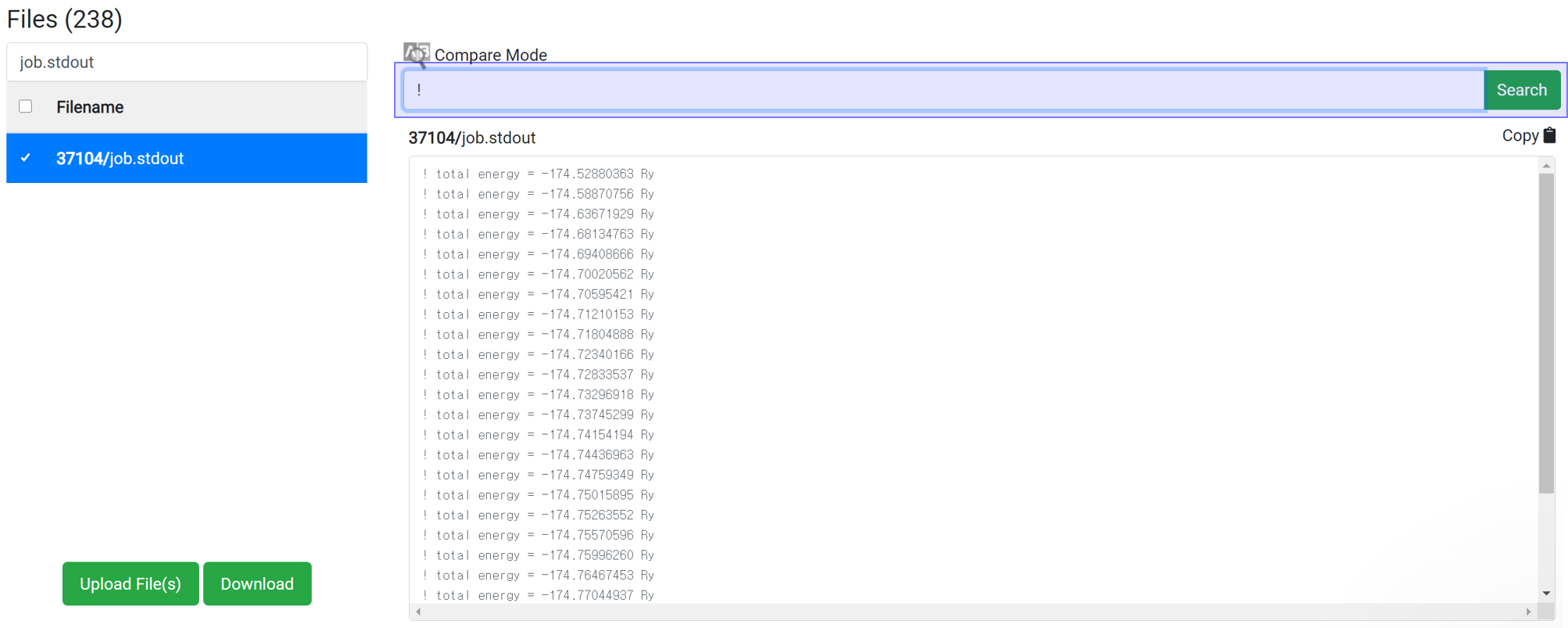Enter ‘!’ in the Find string search box.
In the PWscf calculation, the one 'total energy' value is created when one SCF algorithm finished. So for SCF calculation, this data can be checked after the calculation ends.

This method can apply for the job with at least 1 iteration finished. Enter 'time' in the Find String search box to display the accumulated calculation time. This information is written at the end of one iteration, and the time takes for each iteration is about the same. So you can use it to estimate the total time.
If the time interval is 30 second and 'Max scf steps' is set to 200 and 'Max iteration steps' is set to 100, the maximum consumption time can be estimated to be 30 * 200 * 100 = 600,000 seconds. In most cases, calculations achieve convergence before reach the max steps of course. This is the maximum time just for the case if the calculation does not achieve convergence until the end.Whether the structure can achieve convergence easily can be estimated by searching for 'achieve'. By typing 'achieve' in the search box, you can see how many iterations took to converge at each scf step. Convergence for highly symmetrical structures takes place in about 10 iterations. If convergence does not occur within the set maximum iteration step, increase the maximum iteration step value or change the accuracy of the initial structure or calculation.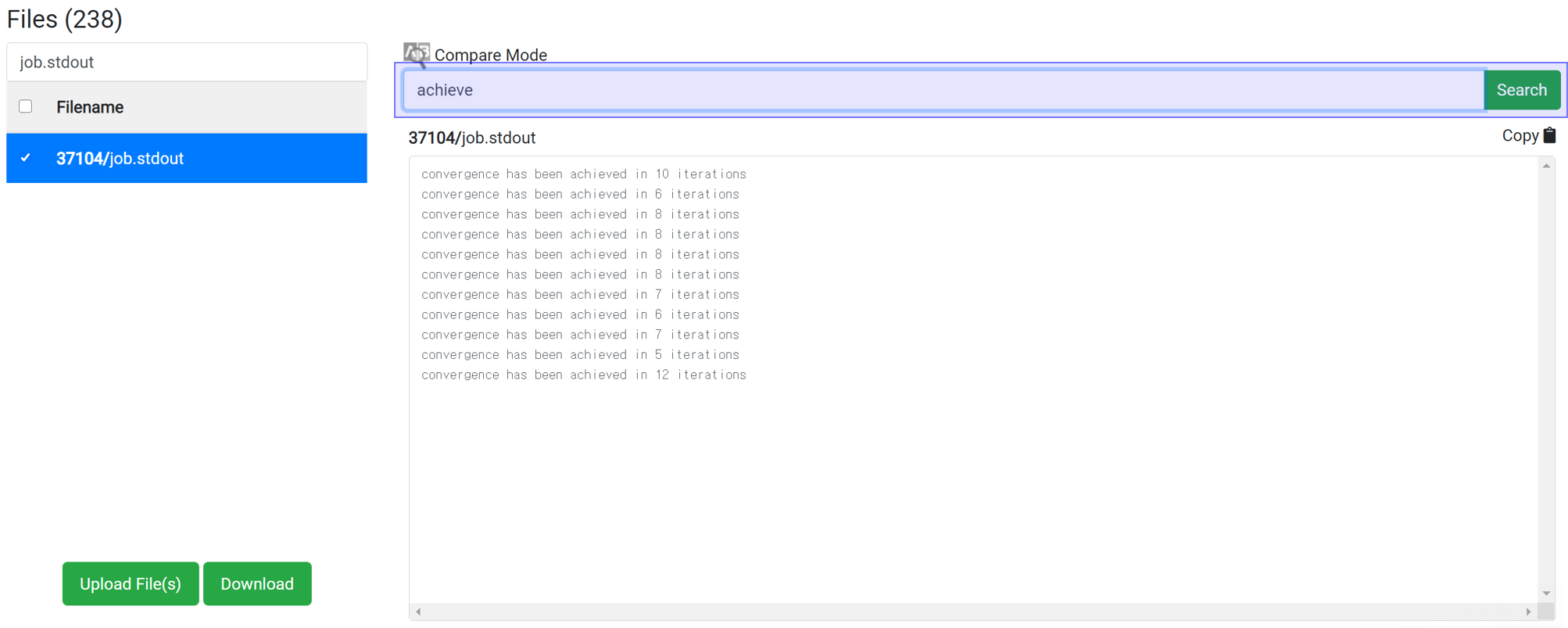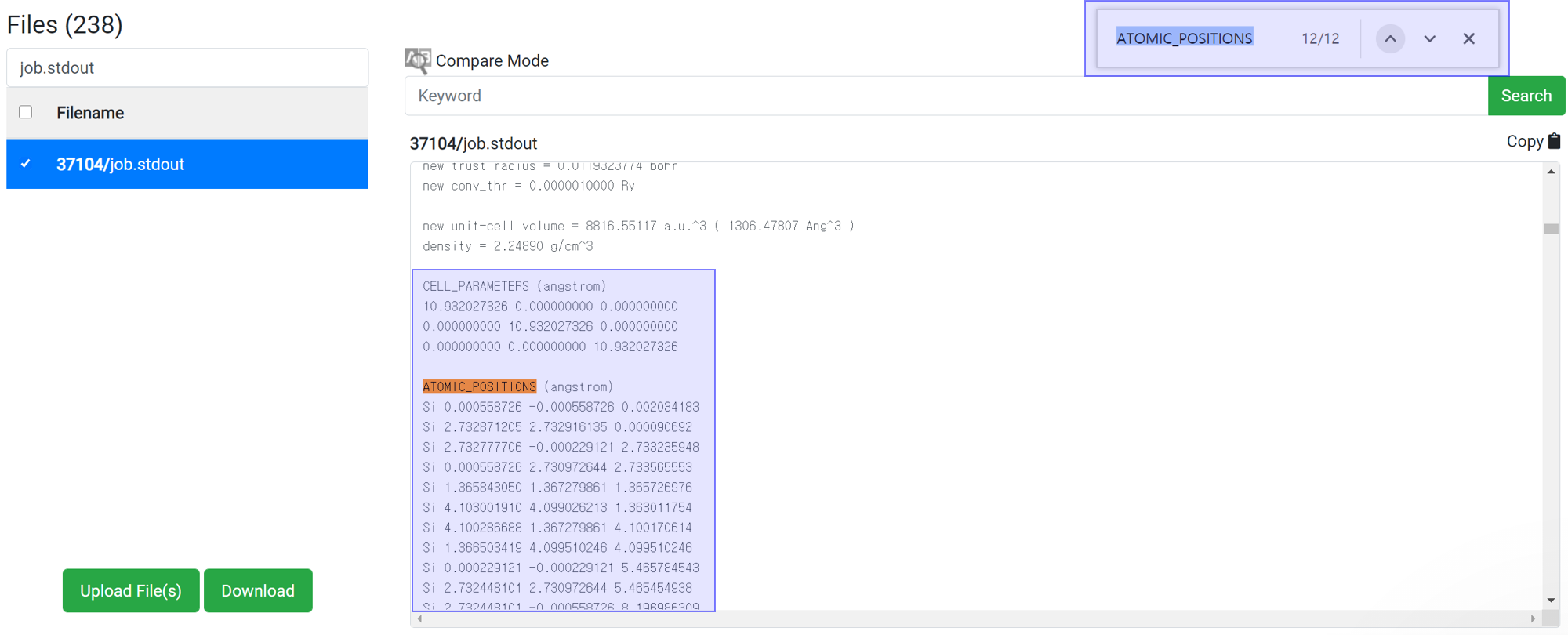Generally, variable cell relaxation calculation takes many calculation time. To check the structure data in the middle of calculation, find the information at the job.stdout.
• Search ‘ATOMIC_’ text at the search box in the browser (ctrl+F).
• Copy the cell parameter and atomic position information.
• And paste that to the ‘EDIT’ menu in the structure builder module.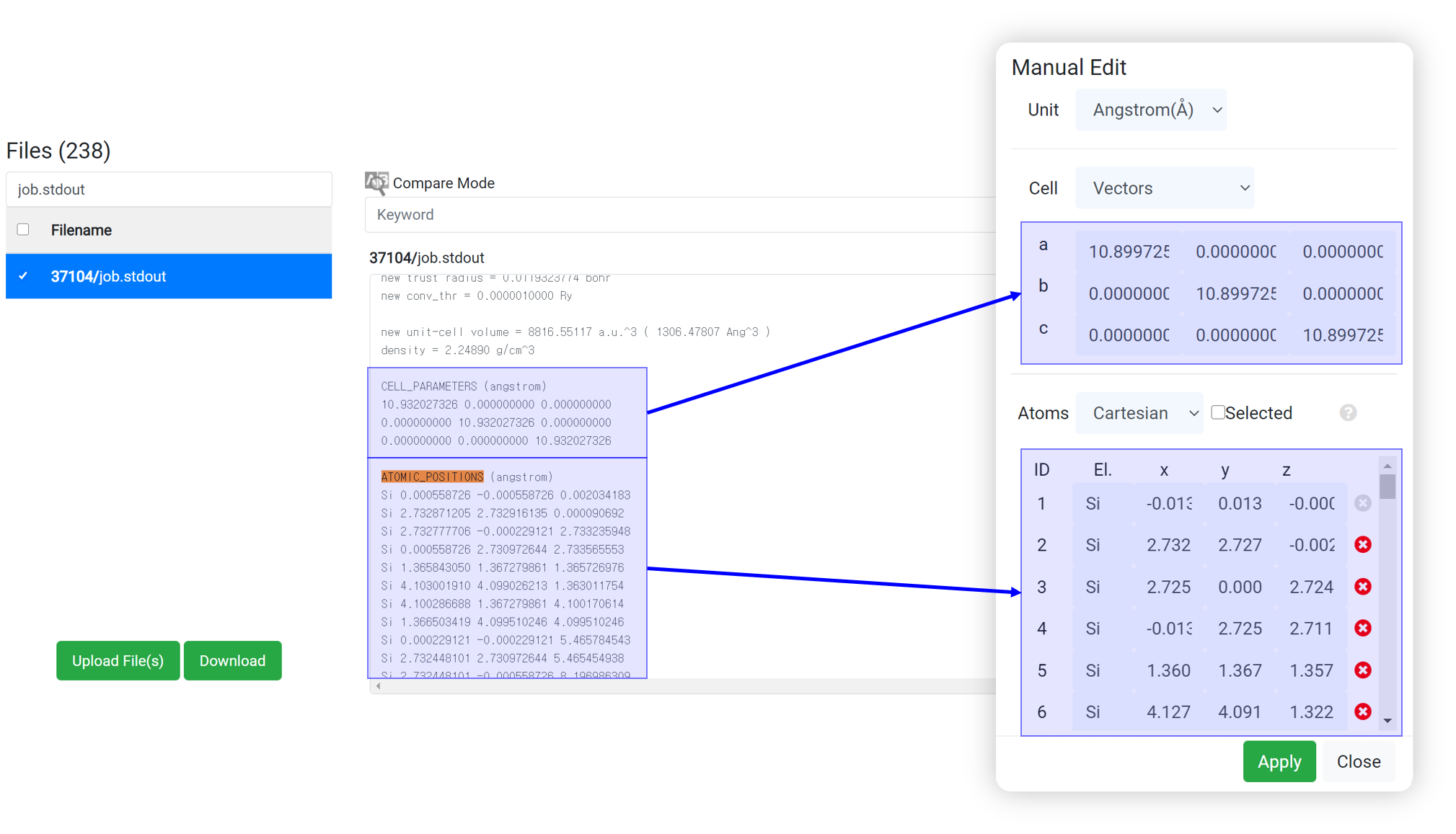Even if 'This has been finished normally' message is displayed in the module, the calculation may not actually have finished normally. Therefore, before checking the result data, check if the following message is at the bottom of 'job.stdout'.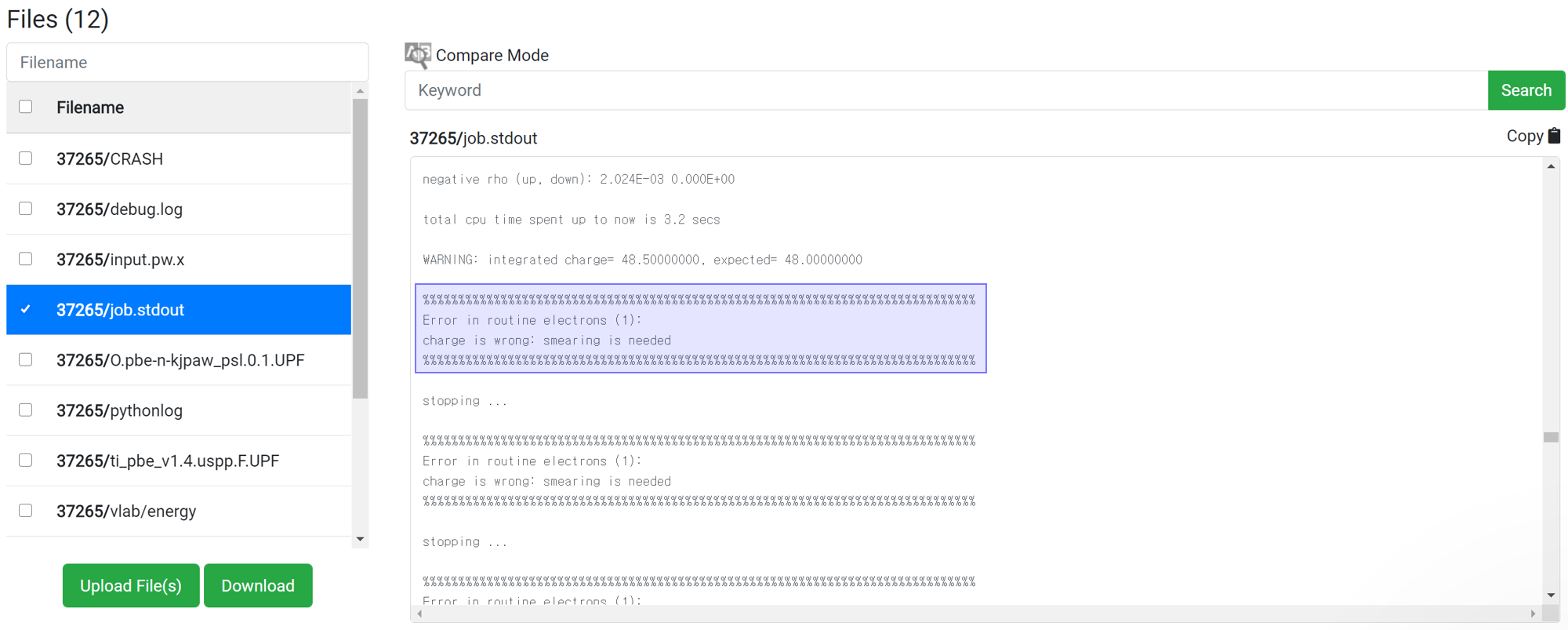For the reason of this error, please refer to Troubleshooting .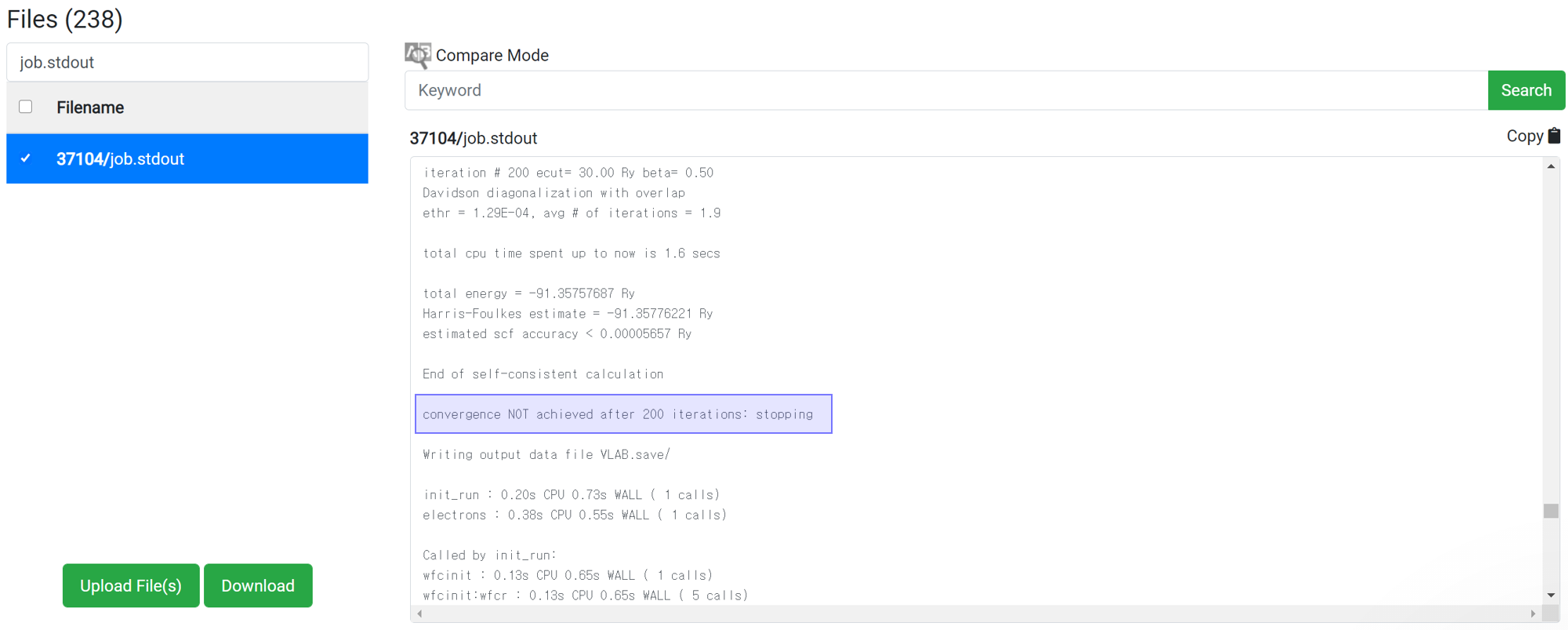For the reason of this error, please refer to Restart .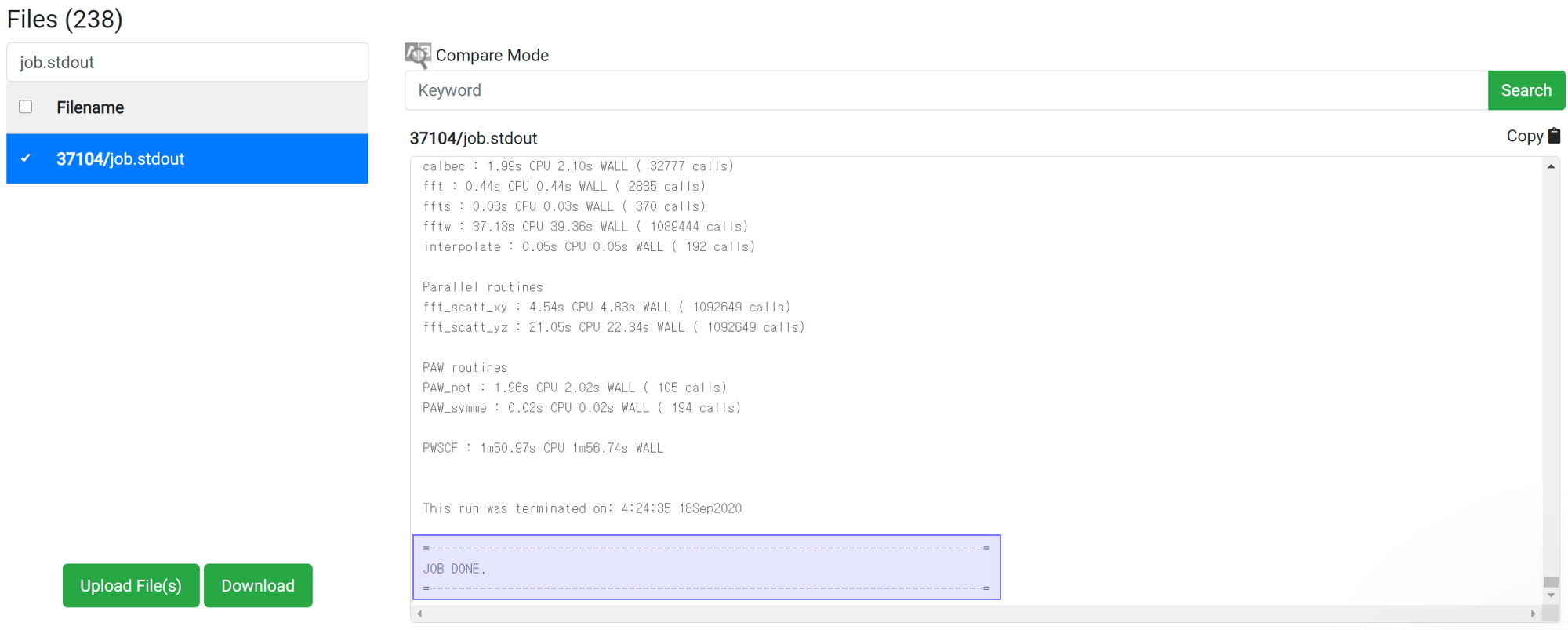If there is no message like ‘Crash’ or ‘Not Achieved’ as above, the job has ended normally.

TemplateFile nameDescription
Common job.stdout The output file of a LAMMPS calculation containing all data related to the LAMMPS calculation.
Common log.lammps The output file of a LAMMPS calculation containing all data related to the LAMMPS calculation.
Common input.lammps The input script of LAMMPS calculation.
Common structure.matsq.lammps The initial structure data of the LAMMPS calculation.
Common #.dat The trajectory file during LAMMPS calculation. The 'Movie' module only works when the trajectory saved in this format.
Calculation Type File Location File name Description
Common EXTBAS Generated basis set file when using custom basis set (external basis set) such as LANL2DZ, def2svp
Common gamess.x.dat Orbital, Energies, Vibrational modes information file
Common gamess.x.inp GAMESS Input file. It contains structural information and designated input parameters
Common job.stdout GAMESS Output file. It contains most of the simulation information, such as energy data.
Common pythonlog File for data parsing
Common vlab/ dos DOS Spectrum and MO Eigenvalues
Common vlab/ energy File for data parsing
Common vlab/ jsondata File for data parsing
Common vlab/ metadata File for data parsing
Common vlab/ moenergies File for data parsing
Common vlab/ order File for data parsing
Common vlab/ status File for data parsing
Common vlab/ thermo File for data parsing
Common vlab/ valence File for data parsing
Common vlab/trajectory #.xyz Trajectory file generated from geometry optimization
NEB gamess.x.trj Trajectory file generated from NEB calculation result
NEB vlab/ neb_data.json File for data parsing
Hessian gamess.x.rst This file need for restart Energy/Gradient/Dipole calculation. It generated when hessian calculation.
BDE vlab/ bde_data.json File for data parsing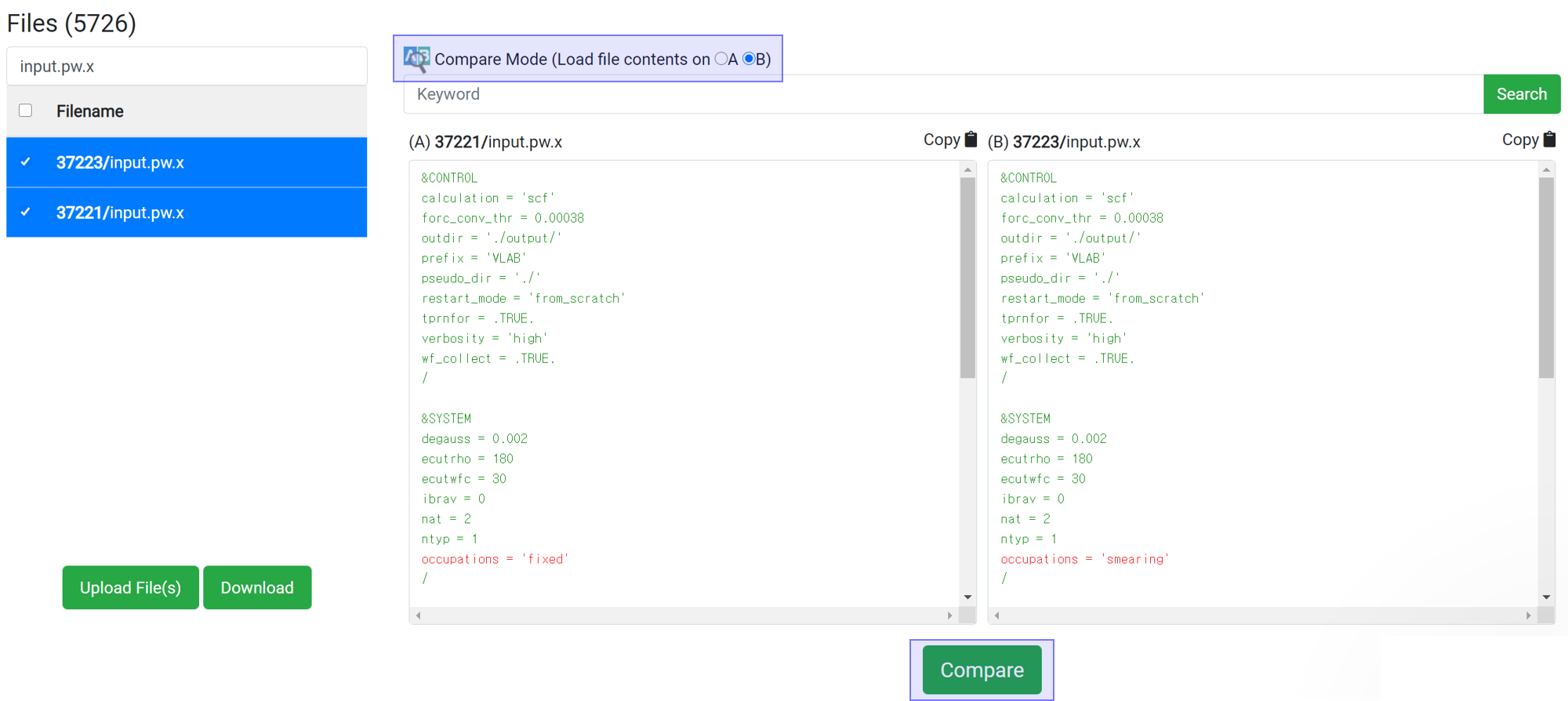If you want to compare two files, press the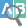button to assign the data to A and B. You can compare the files from different jobs by selecting two jobs in the upside 'Job Data' table. The difference between the two files is displayed in the red-colored text after clicking button.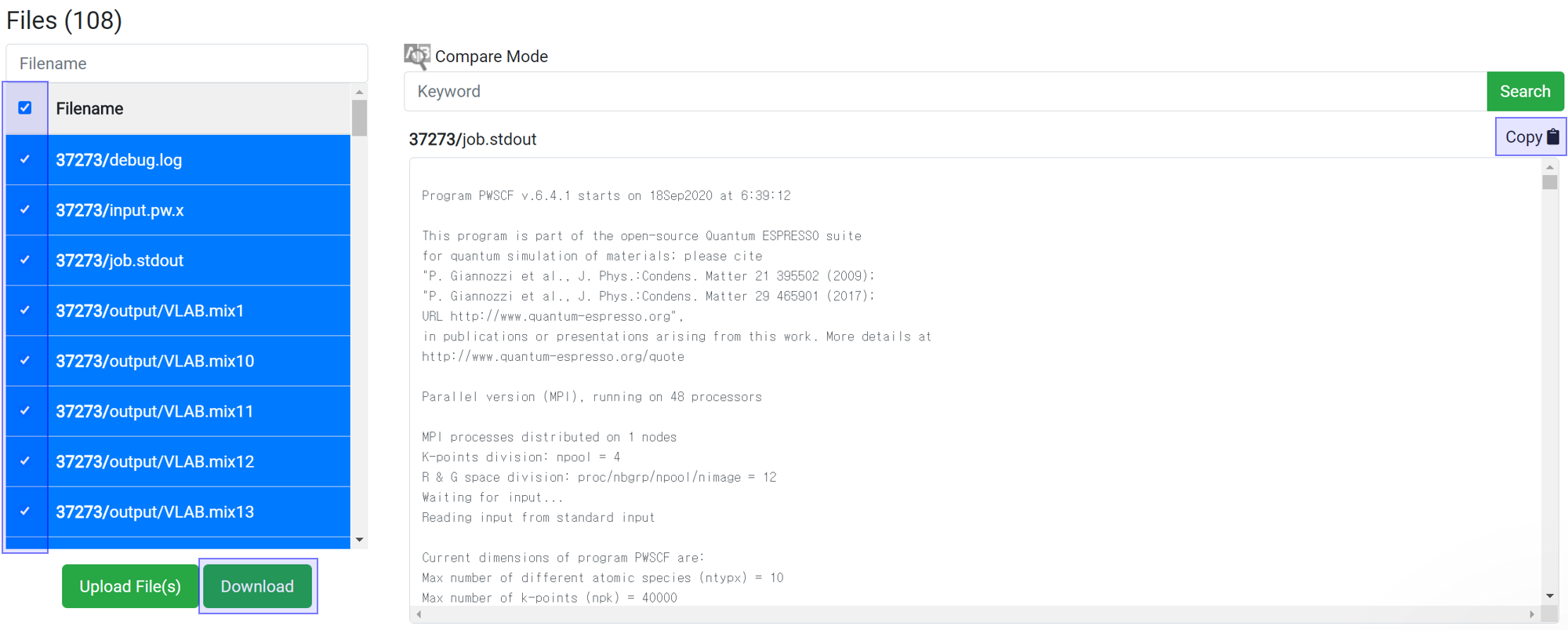You can download data for further processing of the data. Select the desired files and click the button to download the selected file only. Alternatively, you can copy and paste all of the text from the contents window into another program from the 'Copy ' button.